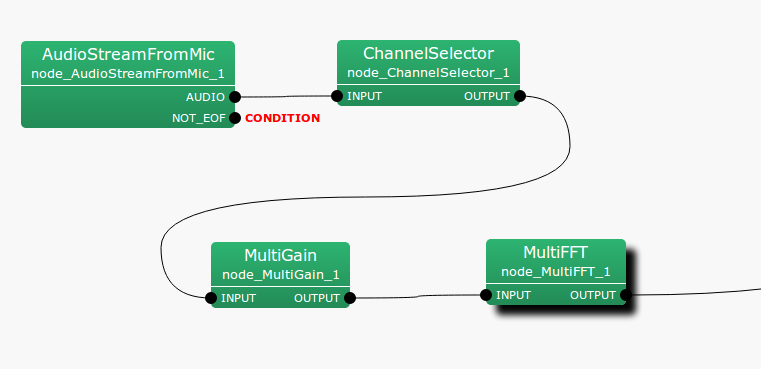## 6.7.8 MultiGain

### 6.7.8.1 Outline of the node

This node regulates gains of input signals.

### 6.7.8.2 Necessary files

No files are required.

### 6.7.8.3 Usage

When to use

This node is used to amplify input signals or modify them so that they cannot be clipped. For example, when speech waveform data quantified by 24 bits are used for inputs on a system built for 16 bits, the MultiGain node is used to decrease the gains by 8 bits.

Typical connection

This node is usually positioned just after AudioStreamFromMic or AudioStreamFromWave , or with ChannelSelector in between.Figure 6.106: Example of a connection of MultiGain

### 6.7.8.4 Input-output and properties of the node

Table 6.78: Parameters of MultiGain
 Parameter name Type Default value Unit Description GAIN 1.0 Gain value

Input

INPUT

: Matrix<float> type. Multichannel speech waveform data (time domain waveform).

Output

OUTPUT

: Matrix<float> type. Multichannel speech waveform data (time domain waveform) for which gains are regulated.

Parameters

GAIN

: float type. A gain parameter. Inputting 1.0 corresponds to outputting the inputs without change.

### 6.7.8.5 Details of the node

Each channel of inputs is output as the values multiplied by the value designated for the GAIN parameter. Note that the inputs are time domain waveforms. For example, when decreasing gains by 40 dB, calculate as follows and designate 0.01.

 $\displaystyle 20 \log x$ $\displaystyle =$ $\displaystyle -40$ (146) $\displaystyle x$ $\displaystyle =$ $\displaystyle 0.01$ (147)